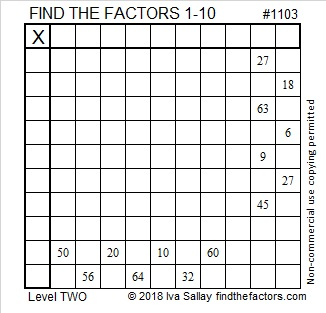# 1103 and Level 2

The fourteen clues you see in this puzzle are all you need to find all the factors from 1 to 10 and complete the multiplication table. Can you find all those factors?Print the puzzles or type the solution in this excel file: 10-factors-1102-1110

Here are some facts about the number 1103:

• 1103 is a prime number.
• Prime factorization: 1103 is prime.
• The exponent of prime number 1103 is 1. Adding 1 to that exponent we get (1 + 1) = 2. Therefore 1103 has exactly 2 factors.
• Factors of 1103: 1, 1103
• Factor pairs: 1103 = 1 × 1103
• 1103 has no square factors that allow its square root to be simplified. √1103 ≈ 33.21144

How do we know that 1103 is a prime number? If 1103 were not a prime number, then it would be divisible by at least one prime number less than or equal to √1103 ≈ 33.2. Since 1103 cannot be divided evenly by 2, 3, 5, 7, 11, 13, 17, 19, 23, 29 or 31, we know that 1103 is a prime number.1103 is the sum of the nineteen prime numbers from 19 to 101.

1103 is palindrome 191 in BASE 29 because 1(29²) + 9(29) + 1(1) = 1103

This site uses Akismet to reduce spam. Learn how your comment data is processed.